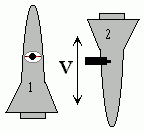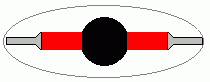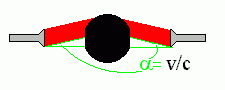## Einstein's Equation E=mc2

I had the great good fortune to come across a rather fascinating essay "The Sextant Equation" [by Peter Galison] about Einstein's famous equation E=mc2. This equations has been derived in many ways, but probably none more simply and elegantly than Einstein's own 1946 derivation which I am attempting to describe third hand, paraphrasing both Einstein's original derivation and Galison's explanatory notes.

What so struck me about this derivation is the incredibly few principles you need to accept. And only one of these principles (the last) is not a cornerstone of classical physics. It should have been possible for almost any physicist in the 19th century to stumble upon this equation but only Einstein early in the 20th century saw it. The principles are:

1. Momentum is conserved (Momentum is mass times velocity. This has been known since Galileo's time.)
2. A moving observer sees light shift angles (This was first seen in stellar aberration in the 18th century.)3. Light has momentum (For example comets tails are driven outwards by sunlight. This has been observed since antiquity.)
4. No body is truly at rest. (Only relative motions can be discussed meaningfully.)

Assume two "spaceships" in free fall are moving with a velocity v1 relative to each other. Aboard one spaceship, an experiment is being conducted. On the other spaceship, nosy snoopers observe the experiment.Both crews agree that a ball is being illuminated by two equally powerful flashlights. On the first ship which is at rest relative to the ball, the effects of the two flashlights seem to cancel each other. No change in the momentum is observed and the ball remains with a zero velocity relative to the first ship. On this ship the crew would say that any momentum imparted by one flashlight is canceled by the other.The snoopers aboard the second ship see things differently. To them, the light seems to swivel ever so slightly upwards2 at an angle a=v/c. They note that ball's velocity remains v, exactly the same as the velocity of the first ship. Since light has momentum, the ball receives a small upward momentum from the upward component of the light. The original momentum of the ball was Mv (its mass times its velocity). Since v is unaltered the new mass must be larger by a small amount m yielding a momentum of (M+m)v.

All we need to do is calculate the upward component of the momentum delivered by the two flashlights. Each flashlight delivers half the total energy E. From classical physics we know that the light's momentum is E/c. Since the upward component of this momentum is a=v/c. Adjusting for the upward momentum we get Ev/c2. All of this is strictly in accordance with classical Newtonian physics, no relativity stuff here.

Adding Ev/c2 to the original momentum Mv increases the ball's momentum. All the added momentum is contained in the increased mass of the ball. Mathematically we now have Mv+Ev/c2=(M+m)v. With some simple algebra3 we get the famous equation E=mc2.

1We shall align v at vertically in our experiment. It could be any angle but a vertical direction avoids messy mathematical terms which cancel out at the end.

2This is exactly what an observer sees when viewing the stars from a moving platform such as the Earth; the stars have an aberration in the direction of motion by an angle v/c where v is the velocity of the Earth.

3Divide each term by by v.
Multiply each term by c2.
Subtract Mc2 from both sides of the equation.

This document was authored by Les Coleman and is subject to Copyrights belonging to Les Coleman. This material may be referenced and reproduced as long as proper attribution is given as specified in Proper Usage Guidelines for Frosty Drew and Related Materials.